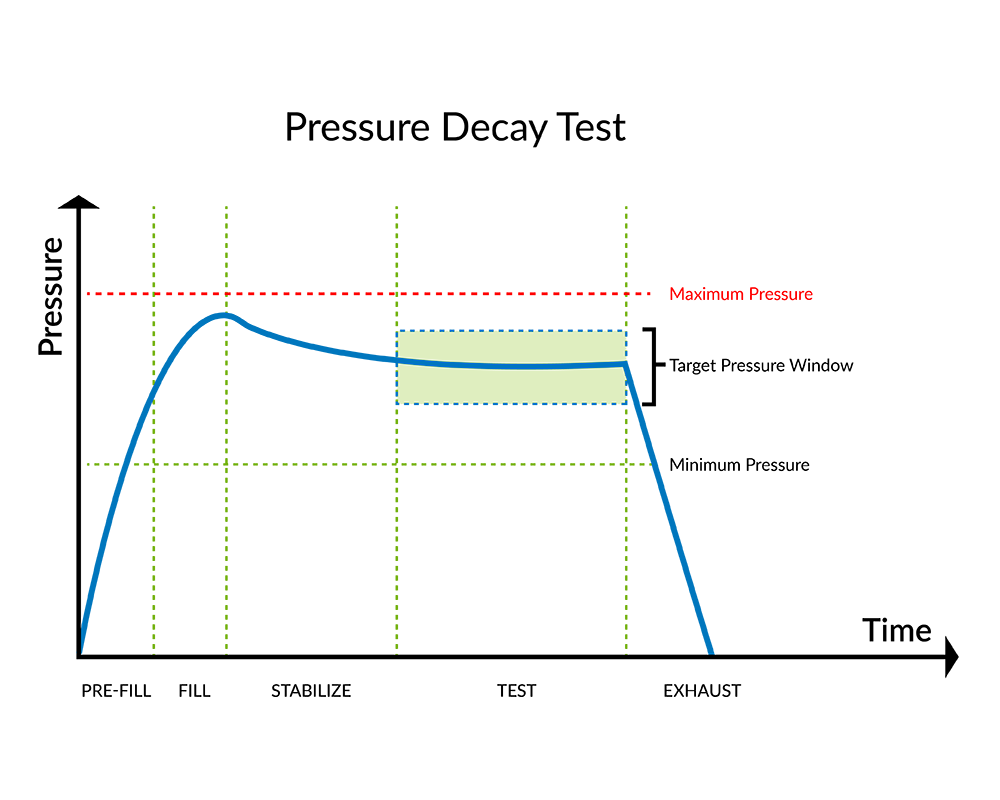# Leak Testing Using Pressure Decay - dP and dP/dT

The Pressure Decay test dP and dP/dT determines pressure loss measured over a fixed time and is presented as the amount of pressure change within the test part volume . The basic operation of a pressure decay test is to fill the test part to a specified target test pressure, isolate the test part circuit from the pressure source, stabilize the pressure and test part, then measure the pressure loss over a defined test time.### Pressure Decay dP and dP/dT Testing is typically used for:

• Applications with very low leak rates
• Stable parts with no volume difference part to part
• Measurable specification when converting from dunk testing to pressure decay testing## How the Pressure Decay dP and dP/dT Leak Test Works

The pressure decay dP and dP/dT test results provide a delta pressure loss as the result of a leak and is reported as dP or dP/dT. The dP test reports the ‘pressure drop’ for the full test time. The dP/dT test measures the pressure drop and reports the ‘calculated pressure drop’ identified as a pressure loss  per one second.

##### Example:  dP and dP/dT test results
 dP Test Test time = 10 sec Measured leak = 1.0 psig Pressure drop = 1 psig in 10 seconds
 dP/dT Test Test time = 10 sec Measured leak = 1.0 psig Pressure drop 1.0 psig / 10 sec  =  0.1 psig per second

As standard, the pressure decay leak test methods can be performed with pressure (P) or vacuum (V) and results identified as dP, dV, dP/dT, or dV/dT.

See if this is the right test method for you.